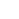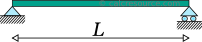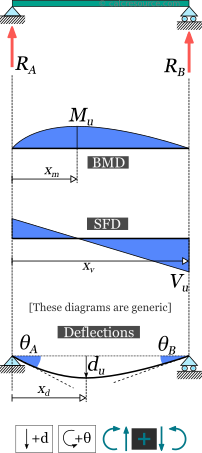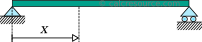Jump to
Share this

## Simply supported beam calculator

This tool calculates the static response of simply supported beams under various loading scenarios. The tool calculates and plots diagrams for these quantities:

• reactions
• bending moments
• transverse shear forces
• deflections
• slopes

Please take in mind that the assumptions of Euler-Bernoulli beam theory are adopted, the material is elastic and the cross section is constant over the entire beam span (prismatic beam).

Units:
 1 2 3 4 Structure L = mcmmmydftin Optional properties, required only for deflection/slope results: E = PakPaMPaGPapsiksiMpsi I = m^4cm^4mm^4ft^4in^4Calculate the moment of inertia of various beam cross-sections, using our dedicated calculators.ADVERTISEMENT
 1 2 3 4 Imposed loading: Uniform distributed loadUniform distr. load (total)Point load (centered)Point loadPoint momentTriangular loadTrapezoidal loadTrapezoidal load (slab)Partial uniform loadPartial triangular loadPartial trapezoidal load
 1 2 3 4 Results: Reactions: RA = kNNkgtlbfkip RB = kNNkgtlbfkip Bending Moment: Mu = kNmNmkg.mt.mlbf.ftlbf.inkip.ftkip.in xm = mcmmmydftin Transverse Shear Force: Vu = kNNkgtlbfkip xv = mcmmmydftin Deflection: du = mcmmmydftin xd = mcmmmydftin Slopes: θA = degradmrad θB = degradmrad1 2 3 4 Request results at a specific point: x = mcmmmydftin M(x) = kNmNmkg.mt.mlbf.ftlbf.inkip.ftkip.in V(x) = kNNkgtlbfkip d(x) = mcmmmydftin θ(x) = degradmradADVERTISEMENT
 1 2 Diagrams kNmNmkg.mt.mlbf.ftlbf.inkip.ftkip.in kNNkgtlbfkip mcmmmydftin degradmrad kN/mN/mkg/mt/mlbf/ftlbf/inkip/ft

ADVERTISEMENT

Share this

## Background

### Introduction

The simply supported beam is one of the most simple structures. It features only two supports, one at each end. One pinned support and a roller support. Both of them inhibit any vertical movement, allowing on the other hand, free rotations around them. The roller support also permits the beam to expand or contract axially, though free horizontal movement is prevented by the other support.

Removing any of the supports or inserting an internal hinge, would render the simply supported beam to a mechanism, that is body the moves without restriction in one or more directions. Obviously this is unwanted for a load carrying structure. Therefore, the simply supported beam offers no redundancy in terms of supports. If a local failure occurs the whole structure would collapse. These type of structures, that offer no redundancy, are called critical or determinant structures. To the contrary, a structure that features more supports than required to restrict its free movements is called redundant or indeterminate structure.

ADVERTISEMENT

#### Assumptions

The static analysis of any load carrying structure involves the estimation of its internal forces and moments, as well as its deflections. Typically, for a plane structure, with in plane loading, the internal actions of interest are the axial force , the transverse shear force and the bending moment . For a simply supported beam that carries only transverse loads, the axial force is always zero, therefore it is often neglected. The calculated results in the page are based on the following assumptions:

• The material is homogeneous and isotropic (in other words its characteristics are the same in ever point and towards any direction)
• The material is linear elastic
• The loads are applied in a static manner (they do not change with time)
• The cross section is the same throughout the beam length
• The deflections are small
• Every cross-section that initially is plane and also normal to the longitudinal axis, remains plane and and normal to the deflected axis too. This is the case when the cross-section height is quite smaller than the beam length (10 times or more) and also the cross-section is not multi layered (not a sandwich type section).

The last two assumptions satisfy the kinematic requirements for the Euler Bernoulli beam theory that is adopted here too.

#### Sign convention

For the calculation of the internal forces and moments, at any section cut of the beam, a sign convention is necessary. The following are adopted here:

1. The axial force is considered positive when it causes tension to the part
2. The shear force is positive when it causes a clock-wise rotation of the part.
3. The bending moment is positive when it causes tension to the lower fiber of the beam and compression to the top fiber.

These rules, though not mandatory, are rather universal. A different set of rules, if followed consistently would also produce the same physical results.

#### Symbols

• : the material modulus of elasticity (Young's modulus)
• : the moment of inertia of the cross-section around the elastic neutral axis of bending
• : the total beam span
• : support reaction
• : deflection
• : bending moment
• : transverse shear force
• : slope

### Simply supported beam with uniform distributed load

The load w is distributed throughout the beam span, having constant magnitude and direction. Its dimensions are force per length. The total amount of force applied to the beam is , where the span length. Either the total force or the distributed force per length may be given, depending on the circumstances.

In the following table, the formulas describing the static response of the simple beam under a uniform distributed load are presented.

ADVERTISEMENT

### Simply supported beam with point force in the middle

The force is concentrated in a single point, located in the middle of the beam. In practice however, the force may be spread over a small area, although the dimensions of this area should be substantially smaller than the beam span length. In the close vicinity of the force application, stress concentrations are expected and as result the response predicted by the classical beam theory is maybe inaccurate. This is only a local phenomenon however. As we move away from the force location, the results become valid, by virtue of the Saint-Venant principle.

In the following table, the formulas describing the static response of the simple beam under a concentrated point force , imposed in the middle, are presented.

### Simply supported beam with point force at a random position

The force is concentrated in a single point, anywhere across the beam span. In practice however, the force may be spread over a small area. In order to consider the force as concentrated, though, the dimensions of the application area should be substantially smaller than the beam span length. In the close vicinity of the force, stress concentrations are expected and as result the response predicted by the classical beam theory maybe inaccurate. This is only a local phenomenon however, and as we move away from the force location, the discrepancy of the results becomes negligible.

In the following table, the formulas describing the static response of the simple beam under a concentrated point force , imposed at a random distance from the left end, are presented.

### Simply supported beam with point moment

In this case, a moment is imposed in a single point of the beam, anywhere across the beam span. In practical terms, it could be a force couple, or a member in torsion, connected out of plane and perpendicular to the beam.

At any case, the moment application area should spread to a small length of the beam, so that it can be successfully idealized as a concentrated moment to a point. Although in the close vicinity the application area, the predicted results through the classical beam theory are expected to be inaccurate (due to stress concentrations and other localized effects), as we move away, the predicted results are perfectly valid, as stated by the Saint-Venant principle.

In the following table, the formulas describing the static response of the simple beam under a concentrated point moment , imposed at a distance from the left end, are presented.

### Simply supported beam with triangular load

The load is distributed throughout the beam span, however, its magnitude is not constant but is varying linearly, starting from zero at the left end to its peak value at the right end. The dimensions of are force per length. The total amount of force applied to the beam is , where the span length.The orientation of the triangular load is important! The formulas presented in this section have been prepared for the case of an ascending load (left-to-right), as shown in the schematic. For a descending load you may mirror the beam, so that its left end (point A) is the least loaded one. The x axis and all results will be mirrored too.

In the following table, the formulas describing the static response of the simple beam under a linearly varying (triangular) distributed load, ascending from the left to the right, are presented.

### Simply supported beam with trapezoidal load

The load is distributed throughout the beam span, having linearly varying magnitude, starting from at the left end, to at the right end. The dimensions of and are force per length. The total amount of force applied to the beam is , where the span length.The values of and can be freely assigned. It is not mandatory for the former to be smaller than the latter. They may take even negative values (one or both of them).

In the following table, the formulas describing the static response of the simple beam under a varying distributed load, of trapezoidal form, are presented.

### Simply supported beam with slab-type trapezoidal load distribution

This load distribution is typical for the beams in the perimeter of a slab. The distribution is of trapezoidal shape, with maximum magnitude at the interior of the beam, while at its two ends it becomes zero. The dimensions of (\w\) are force per length. The total amount of force applied to the beam is , where the span length and , the lengths at the left and right side of the beam respectively, where the load distribution is varying (triangular).

In the following table, the formulas describing the static response of the simple beam under a trapezoidal load distribution, as depicted in the schematic above, are presented.

### Simply supported beam with partially distributed uniform load

The load is distributed to a part of the beam span, with constant magnitude , while the remaining span is unloaded. The dimensions of are force per length. The total amount of force applied to the beam is , where the span length and , the unloaded lengths at the left and right side of the beam, respectively.

In the following table, the formulas describing the static response of the simple beam, under a partially distributed uniform load, are presented.

### Simply supported beam with partially distributed trapezoidal load

The load is distributed to a part of the beam span, having linearly varying magnitude from to , while the remaining span is unloaded. The dimensions of and are force per length. The total amount of force applied to the beam is , where the span length and , the unloaded lengths at the left and right side of the beam respectively.The values of and can be freely assigned. It is not mandatory for the former to be smaller than the latter. They may take even negative values (one or both of them).This is the most generic case. The formulas for partially distributed uniform and triangular loads can be derived by appropriately setting the values of and . Furthermore, the respective cases for fully loaded span, can be derived by setting and to zero.

In the following table, the formulas describing the static response of the simple beam, under a partially distributed trapezoidal load, are presented.

### Related articles

Cantilever beam calculator
Fixed beam calculator
Fixed-pinned beam calculator
Deflections and slopes of simply supported beam
Simply supported beam diagrams
Support reactions of simply supported beam
Column buckling calculator
Moments of Inertia Table
All calculators on Statics

Liked this page? Share it with friends!

ADVERTISEMENT